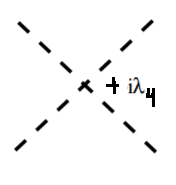# Feynman diagram for interactions in $\phi^3$ complex scalar field theory

I recently encountered the Lagrangian density

$$\mathcal L = \partial_\mu \phi^\dagger \partial^\mu \phi - m^2 \phi^\dagger \phi - \frac{\lambda}{3!} \phi^3 - \frac{\lambda}{3!} \phi^{\dagger 3}.$$

I thought I understood complex scalar field theory, but after trying to figure out this one I've realized that I obviously don't. In standard complex $$\phi^4$$ theory, the interaction term is $$\frac{\lambda_4}{4!}(\phi^\dagger \phi)^2$$. As such, I interpret a vertex, e.g.to mean that two $$\phi$$'s are coming into the vertex, while two $$\phi$$'s are leaving the vertex. So I interpret the complex conjugation of the $$\phi$$ to indicate negating the momentum. Thus $$(\phi^\dagger \phi)^2 = \phi^\dagger \phi^\dagger \phi \phi$$ would have two particles with incoming momentum and two particles with outgoing momentum.

However, in the Lagrangian I posted above, there are two interaction terms $$\frac{\lambda}{3!}\phi^3$$ and $$\frac{\lambda}{3!}\phi^{\dagger 3}$$. What does this mean? In my interpretation above, it would indicate a vertex with either three particles incoming or three particles outgoing. But I'm struggling to understand if that is the case.

How would one write down the Feynman vertex rules for this interaction? When I try to write out $$\langle f \vert T \exp\left[-i \int \frac{\lambda}{3!}\phi^{\dagger 3}(x) d^4x \right] \vert i \rangle$$ for example, I find that this gives a vertex term of $$-i\lambda$$ if $$\vert i \rangle = \vert \phi \phi \phi\rangle$$ and $$\vert f \rangle = \vert 0 \rangle$$, and otherwise $$0$$. But I'm assuming this is incorrect as it means that we start with three $$\phi$$'s and end with zero.

Any help would be appreciated, thanks!

Edit

It seems that with this Lagrangian, we must keep track of two particles $$\phi$$ and $$\phi^\dagger$$. In particular, unlike in real $$\phi^3$$ theory, it seems to me that we don't have vertices of the form $$\phi \to 2 \phi$$, but instead have $$\phi \to 2 \phi^\dagger$$ and $$\phi^\dagger \to 2 \phi$$. So for example a vertex may look likeIf I were to look for the vertex correction, real $$\phi^3$$ theory, I would just add a loop by connecting the two incoming legs. But is this true for complex $$\phi^3$$ theory? When I add such a line, the vertex becomes $$2\phi^\dagger \to \phi^\dagger$$ instead of $$2\phi \to \phi^\dagger$$,Is this correct? Am I correct in saying that vertex corrections for complex $$\phi^3$$ theory require more complicated diagrams?

• as a hint, can you spot any symmetry in the "usual" $|\phi|^4$ theory that is not present in the $\phi^3+(\phi^\dagger)^3$ theory? Mar 2, 2021 at 16:13
• $\phi \to e^{i\theta}\phi$? What does one do with this?
– Joe
Mar 2, 2021 at 16:35
• Your intuition that you need 2 $\phi$'s incoming and 2 outgoing particles is perhaps related to this symmetry, which requires charge is conserved at each vertex. For this theory, there's no problem with (say) 2 $\phi$ particles annihilating and producing one $\phi$. Mar 2, 2021 at 16:42

Why don't you expand these using creation and annihilation operators (standard textbook procedure) and plug then compute $$S$$ matrix elements the old fashion way.
$$S = \langle\text{out}| U |\text{in}\rangle$$
• Yes for a complex scalar there are two sets of creation/annihilation operators. Call them $a/a^{\dagger}$ and $b/b^{\dagger}$. I recommend looking at Peskin and Schroeder for the relevant expansions in terms of these operators and how to compute S matrix elements Mar 2, 2021 at 17:00